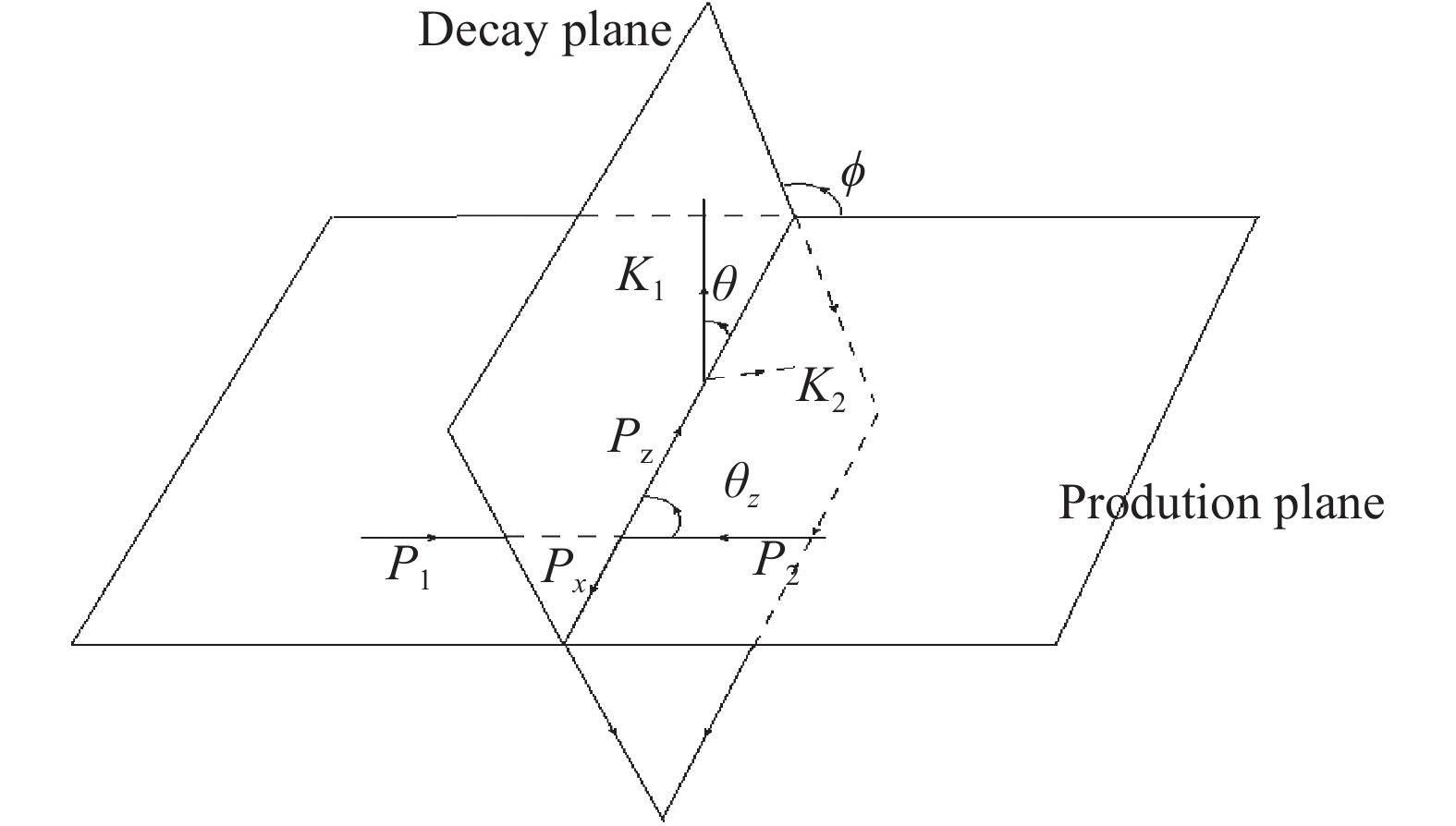# Angular distribution coefficients of Z (W) boson produced in ${{e^+e^-}}$collisions at ${{\sqrt{s}=240}}$GeV• At the designed circular electron-positron collider (CEPC), similar to the hadron collider, the angular distribution coefficients of the decay lepton pair from the produced Z(W) boson in $e^+ e^-$collisions are predicted. Their dependence on cos$\theta_Z$(cos$\theta_W$) are presented in four different polarization frames. Furthermore, the value of the angular distribution coefficients in different bins of cos$\theta_Z$are presented in the C-S frame. In comparison with the case at the hadron collider, better accurate measurement for Z(W) is expected because there is less background, and W could be reconstructed from its leptonic decay channel. This works provides a method to precisely test the electroweak production mechanism or some effect induced from new physics in the future measurements at the CEPC.
••Get Citation
Yu-Dong Wang and Jian-Xiong Wang. Angular distribution coefficients of Z(W) boson produced in ${{e^+e^-}}$collisions at ${{\sqrt{s}=240}}$GeV[J]. Chinese Physics C. doi: 10.1088/1674-1137/43/4/043102
Yu-Dong Wang and Jian-Xiong Wang. Angular distribution coefficients of Z(W) boson produced in ${{e^+e^-}}$collisions at ${{\sqrt{s}=240}}$GeV[J]. Chinese Physics C.Milestone
Received: 2018-12-24
Article Metric

Article Views(338)
PDF Downloads(47)
Cited by(0)
Policy on re-use
To reuse of Open Access content published by CPC, for content published under the terms of the Creative Commons Attribution 3.0 license (“CC CY”), the users don’t need to request permission to copy, distribute and display the final published version of the article and to create derivative works, subject to appropriate attribution.
###### 通讯作者: 陈斌, bchen63@163.com
• 1.

沈阳化工大学材料科学与工程学院 沈阳 110142

Title:
Email:

## Angular distribution coefficients of Z (W) boson produced in ${{e^+e^-}}$collisions at ${{\sqrt{s}=240}}$GeV

• Institute of High Energy Physics, Chinese Academy of Sciences, Beijing 100049, China
• School of Physics, University of Chinese Academy of Sciences, Beijing 100049, China

Abstract: At the designed circular electron-positron collider (CEPC), similar to the hadron collider, the angular distribution coefficients of the decay lepton pair from the produced Z(W) boson in $e^+ e^-$collisions are predicted. Their dependence on cos$\theta_Z$(cos$\theta_W$) are presented in four different polarization frames. Furthermore, the value of the angular distribution coefficients in different bins of cos$\theta_Z$are presented in the C-S frame. In comparison with the case at the hadron collider, better accurate measurement for Z(W) is expected because there is less background, and W could be reconstructed from its leptonic decay channel. This works provides a method to precisely test the electroweak production mechanism or some effect induced from new physics in the future measurements at the CEPC.

### HTML1.   Introduction2.   The angular distribution of the lepton pair3.   Cross section for Z(W) boson production4.   Angular distribution coefficients of Z(W) production processes5.   Summary and conclusion
Reference (29)
PDF查看关注分享

Top

### 目录/DownLoad:  Full-Size Img  PowerPoint